# Help - csens.org

## The basics

1. Start by selecting Source group
-Then select Source
-Then you may select geographical region
2. You may select start and stop year in the “From” and “To” input fields
3. You may select operations to be done on the data. These are explained below
4. Click “Plot graph”, and voila, a nice line graph appears.

## The interactive controls

Some of the controls are interactive, that means that they take effect on the plot immediately after they are changed. You don’t have to click the “Plot graph” button for those. The interactive controls are those just below the plot, and those below the operations in the sources selection.

You may add as many data series as you want and compare them all in one plot.

## The operations (value)

Moving average (Months) - This operation calculate the average of the number of months specified in the value field to the right. Some series are annual and for those the value field must be at least 24 before the operation has any effect. Monthly series will be smoothened if the value is 2 or greater
Low-pass filter(Months) – This operation calculate a weighted average of the number of months specified in the value field to the right. The difference from moving average is that months in the center of the averaged area carry more weight than the first and last months in the interval. See example below.
Stepwise mean (Months) - This operation calculates an average value and plots this as a horizontal line for the number of months specified.
Decadal mean (None) – This operation calculates the average value for each decade and plots this as a horizontal line. No value shall be specified for this operation.
Scale (Number) – This operation multiply all numbers by the given value. This can be useful if you want to compare two series that are given in different units. See examples below.
Derivative (Months) – This operation calculate the difference between the current value and the value the specified number of months before. This operation is useful for calculating the growth rate, see example below.

## Examples

### Illustration of the effect of smoothing filters

Three plots are shown of RSS satellite data. This is 1. Raw monthly data, 2. 60 month floating average 3. 60 month Lowpass filter.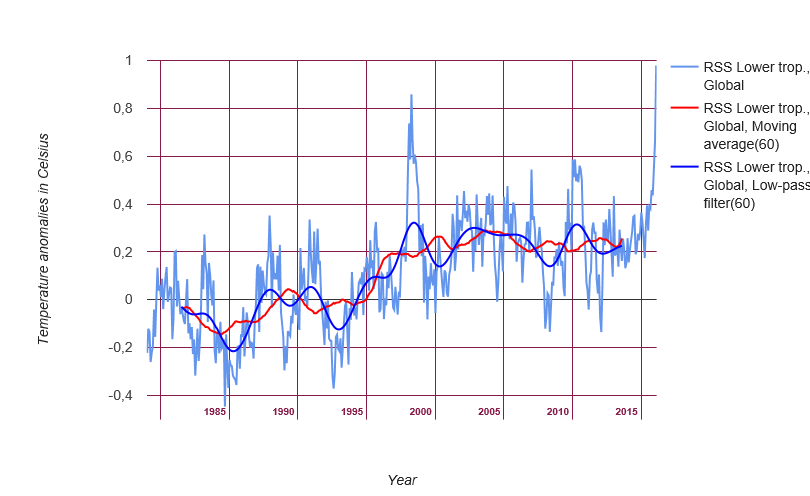Click for larger picture

Even tough moving average is the most widely used smoothing technique, the low-pass filter generally gives a much better representation of the data. This is illustrated in the plot above. The moving average, red line, for instance give a top in 2000 and another in 2008 when the raw data show low values. These tops are artefacts due to high values in the beginning and end of the smoothing interval.

The low-pass filer, blue line, gives a smoothing which better follow the curves in the raw data. The low pass filter here is a “Blackman filter”, which has a weighting of the input as seen in the bell shaped figure below.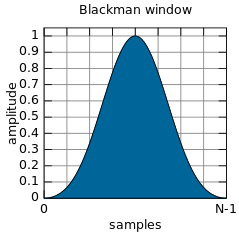Click for larger picture

### Illustration of annual growth by using the "Derivative" operation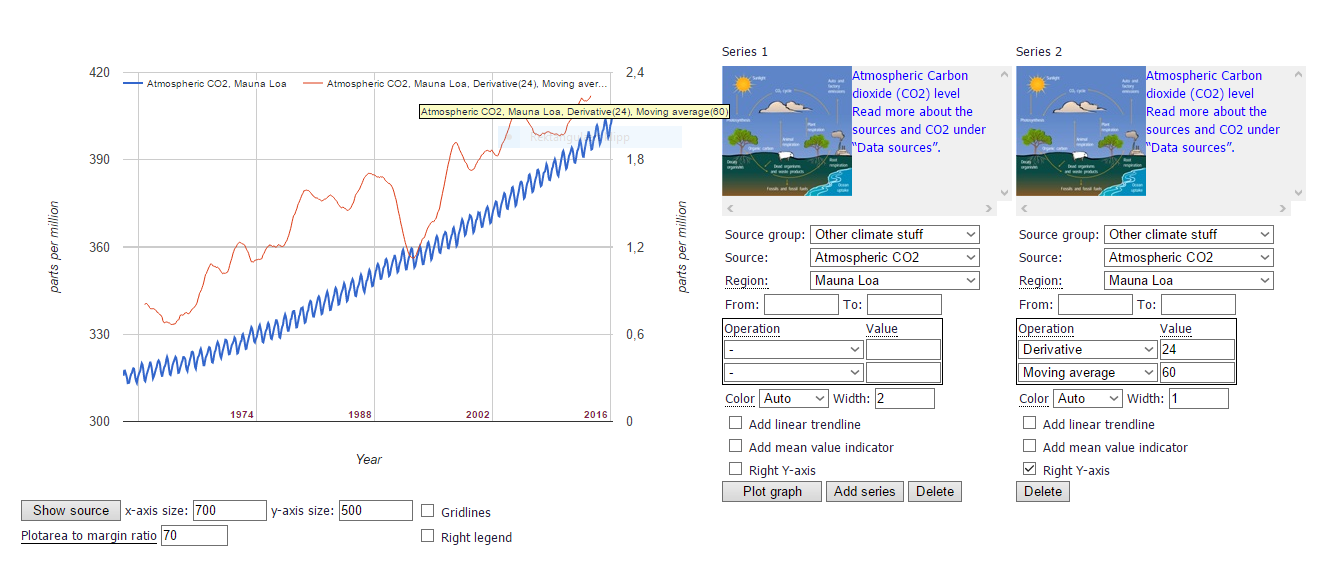Click for larger picture

The blue line show the CO2 level in ppm and the red line show the derivative over 24 months then smoothed with a low-pass filter. The blue line has an annual zig zag pattern due to seaonal changes between ocean and atmosphere, and a weak upward curvature. The curvature shows that the growth rate is increasing, but the actual growth rate is not easily seen by the blue line.

The blue line follow the scale on the left axis and the red line the scale on the right axis.

We can see from the blue plot that the CO2 level has increased from appoximately 315 ppm in 1960 to 400 ppm in 2015 The red plot shows that the annual growth rate started on less than 1.0 ppm/year in the 1960-ies and has increased to more than 2.0 ppm/year after 2000.

### Use of Decadal mean and moving average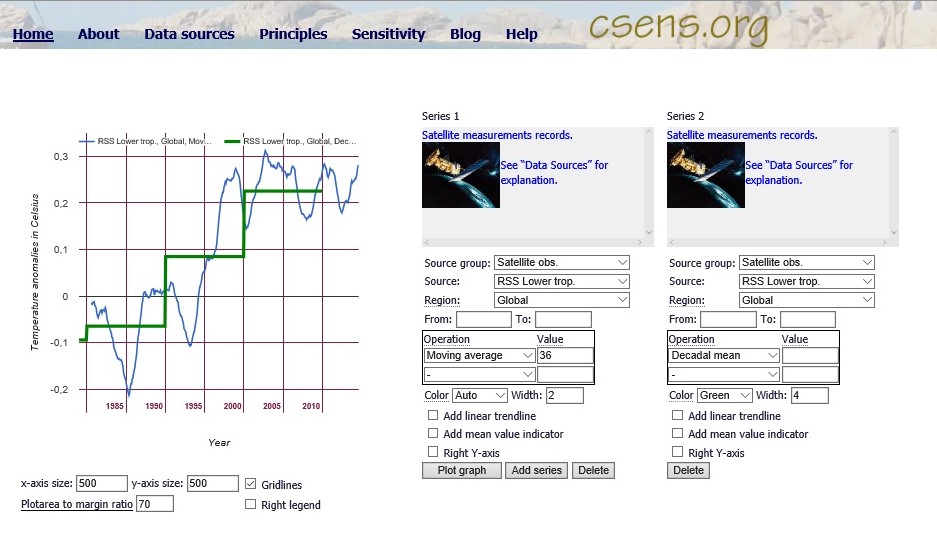Click for larger picture

Moving average over 36 months and Decadal mean are used on the same source to illustrate the long term trend of the Satellite records.

### Use of "Scale -1" to turn the direction of a plot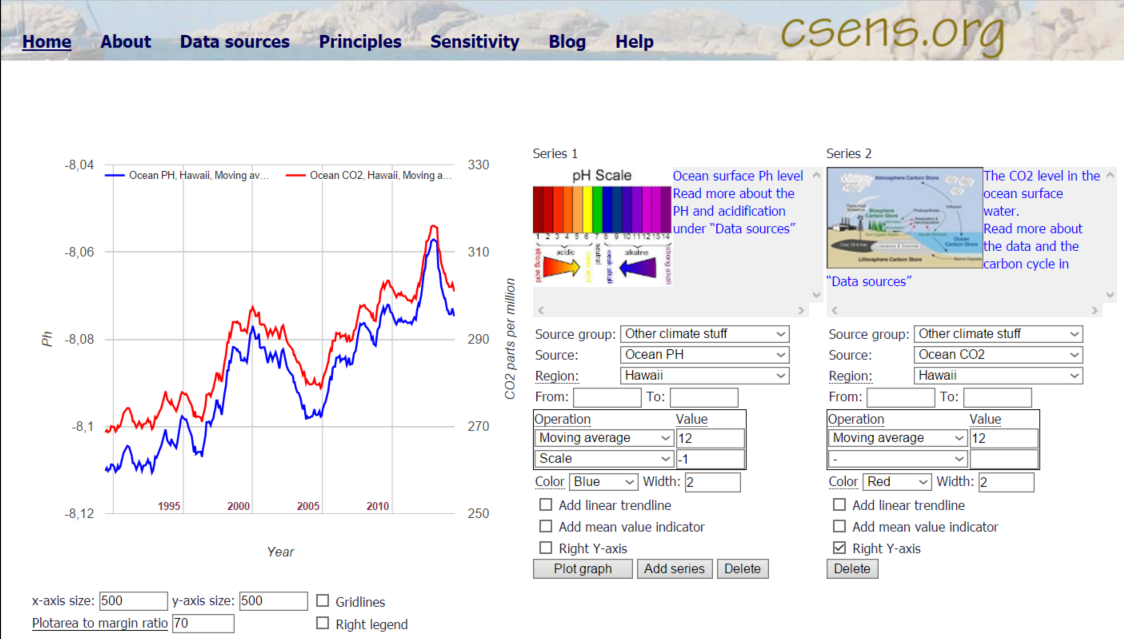Click for larger picture

The Ph and CO2 level in the ocean surface water are headed in opposite directions. By using the "Scale -1" operation one can turn the direction of a graph. By choosing "Right axis" on one of the plots the will be shown alongside each other, and the correlation between the graphs is easily spotted.